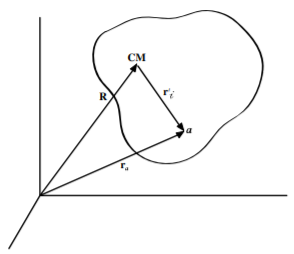$$\require{cancel}$$

# 2.7: Center of Mass of a Many-Body System

$$\newcommand{\vecs}{\overset { \rightharpoonup} {\mathbf{#1}} }$$ $$\newcommand{\vecd}{\overset{-\!-\!\rightharpoonup}{\vphantom{a}\smash {#1}}}$$$$\newcommand{\id}{\mathrm{id}}$$ $$\newcommand{\Span}{\mathrm{span}}$$ $$\newcommand{\kernel}{\mathrm{null}\,}$$ $$\newcommand{\range}{\mathrm{range}\,}$$ $$\newcommand{\RealPart}{\mathrm{Re}}$$ $$\newcommand{\ImaginaryPart}{\mathrm{Im}}$$ $$\newcommand{\Argument}{\mathrm{Arg}}$$ $$\newcommand{\norm}{\| #1 \|}$$ $$\newcommand{\inner}{\langle #1, #2 \rangle}$$ $$\newcommand{\Span}{\mathrm{span}}$$ $$\newcommand{\id}{\mathrm{id}}$$ $$\newcommand{\Span}{\mathrm{span}}$$ $$\newcommand{\kernel}{\mathrm{null}\,}$$ $$\newcommand{\range}{\mathrm{range}\,}$$ $$\newcommand{\RealPart}{\mathrm{Re}}$$ $$\newcommand{\ImaginaryPart}{\mathrm{Im}}$$ $$\newcommand{\Argument}{\mathrm{Arg}}$$ $$\newcommand{\norm}{\| #1 \|}$$ $$\newcommand{\inner}{\langle #1, #2 \rangle}$$ $$\newcommand{\Span}{\mathrm{span}}$$

A finite sized body needs a reference point with respect to which the motion can be described. For example, there are 8 corners of a cube that could server as reference points, but the motion of each corner is complicated if the cube is both translating and rotating. The treatment of the behavior of finite-sized bodies, or many-body systems, is greatly simplified using the concept of center of mass. The center of mass is a particular fixed point in the body that has an especially valuable property; that is, the translational motion of a finite sized body can be treated like that of a point mass located at the center of mass. In addition the translational motion is separable from the rotational-vibrational motion of a many-body system when the motion is described with respect to the center of mass. Thus it is convenient at this juncture to introduce the concept of center of mass of a many-body system.Figure $$\PageIndex{1}$$: Position vector with respect to the center of mass.

For a many-body system, the position vector $$\mathbf{r}_i$$, defined relative to the laboratory system, is related to the position vector $$\mathbf{r}_i^\prime$$ with respect to the center of mass, and the center-of-mass location $$\mathbf{R}$$ relative to the laboratory system. That is, as shown in Figure $$\PageIndex{1}$$

$\label{2.22}\mathbf{r}_i = \mathbf{R} +\mathbf{r}^\prime_i$

This vector relation defines the transformation between the laboratory and center of mass systems. For discrete and continuous systems respectively, the location of the center of mass is uniquely defined as being where

$\label{eq:Center of mass definition}\tag{Center of mass definition}\sum^n_i m_i \mathbf{r}^\prime_i = \int \mathbf{r}^\prime \rho dV = 0$

Define the total mass

$\label{eq:Total mass}\tag{Total mass}M=\sum_i^n m_i = \int_{body}\rho dV$

The average location of the system corresponds to the location of the center of mass since $$\frac{1}{M}\sum_im_i\mathbf{r}^\prime_i = 0$$ that is

$\label{eq:2.23}\frac{1}{M}\sum_im_i\mathbf{r}_i =\mathbf{R} + \frac{1}{M}\sum_im_i\mathbf{r}^\prime_i = \mathbf{R}$

The vector $$\mathbf{R}$$ which describes the location of the center of mass, depends on the origin and coordinate system chosen. For a continuous mass distribution the location vector of the center of mass is given by

$\label{eq:2.24}\mathbf{R}=\frac{1}{M}\sum_im_i\mathbf{r}_i=\frac{1}{M}\int\mathbf{r}\rho dV$

The center of mass can be evaluated by calculating the individual components along three orthogonal axes.

The center-of-mass frame of reference is defined as the frame for which the center of mass is stationary. This frame of reference is especially valuable for elucidating the underlying physics which involves only the relative motion of the many bodies. That is, the trivial translational motion of the center of mass frame, which has no influence on the relative motion of the bodies, is factored out and can be ignored. For example, a tennis ball $$(0.06 \ kg )$$ approaching the earth $$( 6 \times 10^{24} \ kg )$$ with velocity $$v$$ could be treated in three frames, (a) assume the earth is stationary, (b) assume the tennis ball is stationary, or (c) the center-of-mass frame. The latter frame ignores the center of mass motion which has no influence on the relative motion of the tennis ball and the earth. The center of linear momentum and center of mass coordinate frames are identical in Newtonian mechanics but not in relativistic mechanics as described in chapter $$17.4.3$$.

This page titled 2.7: Center of Mass of a Many-Body System is shared under a CC BY-NC-SA 4.0 license and was authored, remixed, and/or curated by Douglas Cline via source content that was edited to the style and standards of the LibreTexts platform; a detailed edit history is available upon request.